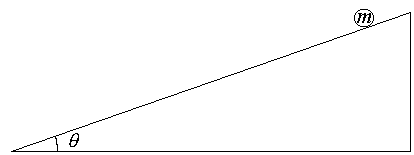First Name: ___________________ Last Name: ____________________ Section: _________

March 27, 1998 Physics 103

Exam 2

Print your name and section clearly on all five pages. (If you do not know your section number, write your TAs name.) Show all work in the space immediately below each problem. Your final answer must be placed in the box provided. Problems will be graded on reasoning and intermediate steps as well as on the final answer. Be sure to include units wherever necessary, and the direction of vectors. Each problem is worth 25 points. In doing the problems, try to be neat. Check your answers to see that they have the correct dimensions (units) and are the right order of magnitudes. You are allowed one 8½ x 11" sheet of notes and no other references. The exam lasts exactly 50 minutes.

(Do not write below)

SCORE:

Problem 1: __________

Problem 2: __________

Problem 3: __________

Problem 4: __________

TOTAL: ___________

First Name: ___________________ Last Name: ____________________ Section: _________

1. A 1000-kg airplane with a 200-horsepower engine (1 horsepower = 746 W) is capable of level flight at a speed of 50 m/s at 65% of full power.

a. What thrust does the engine develop under the above conditions? (9 pts.)

b. If the power is increased to 100% and the speed remains the same, at what rate (m/s) does the airplane gain altitude? (8 pts.)

c. If the engine quits, how long does it take for the plane to lose 1000 m of altitude if the speed remains the same? (8 pts.)

First Name: ___________________ Last Name: ____________________ Section: _________

2. A 4000-pound truck traveling 30 MPH collides head-on with a stationary 2000-pound car. After the collision, the truck is traveling 15 MPH.

a. What is the speed of the car after the collision (in MPH)? (10 pts.)

b. What fraction of the mechanical energy is lost in the collision? (15 pts.)

First Name: ___________________ Last Name: ____________________ Section: _________

3. The planet Neptune has a moon Triton that is 3.6 x 108 m from the planet and orbits Neptune in a circle once every 6 earth days.

1. Calculate the orbital speed of Triton. (10 pts.)

b. Calculate the mass of Neptune. (15 pts.)

First Name: ___________________ Last Name: ____________________ Section: _________4. A ball of mass m, radius r, and moment of inertia 0.4mr2 is released from rest and rolls down a plane inclined at an angle of q = 20 degrees as shown.

a. Calculate the acceleration of the center of mass of the ball. (15 pts.)

b. What minimum coefficient of friction is required to keep the ball from slipping? (10 pts.)